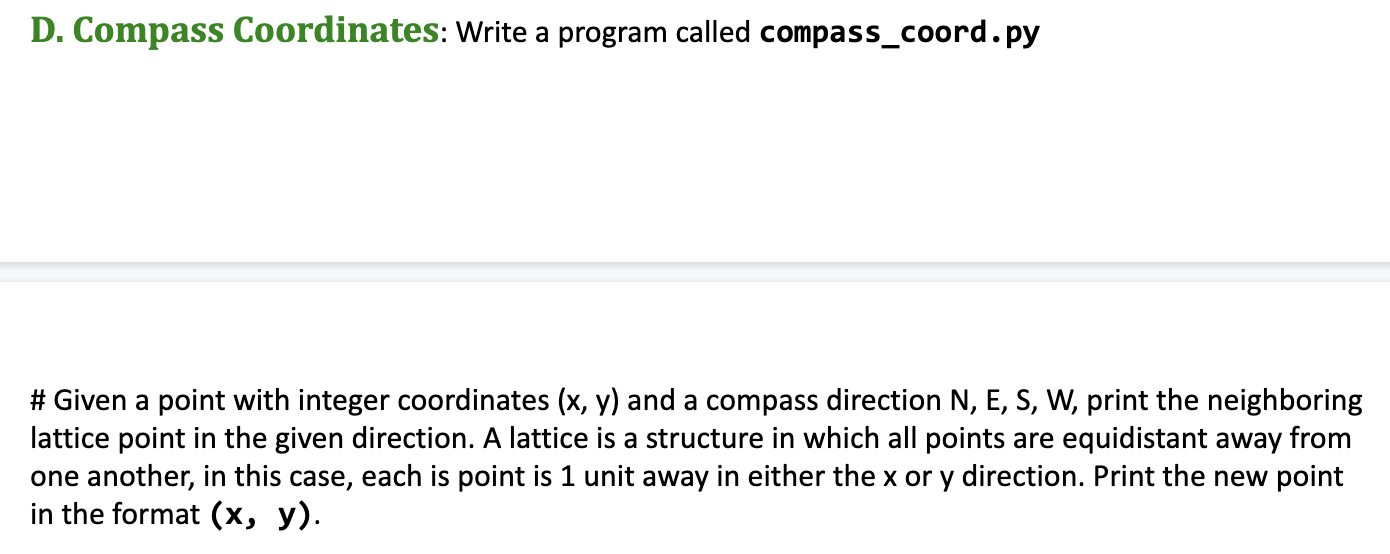# D. Compass Coordinates: Write a program called compass_coord.py#Given a point with integer coordinates (x, y) and a compass direction N, E, S, W, print the neighboringlattice point in the given direction. A lattice is a structure in which all points are equidistant away fromone another, in this case, each is point is 1 unit away in either the x or y direction. Print the new pointin the format (x, y).

Questionhelp_outlineImage TranscriptioncloseD. Compass Coordinates: Write a program called compass_coord.py #Given a point with integer coordinates (x, y) and a compass direction N, E, S, W, print the neighboring lattice point in the given direction. A lattice is a structure in which all points are equidistant away from one another, in this case, each is point is 1 unit away in either the x or y direction. Print the new point in the format (x, y). fullscreen
check_circleExpert Solution
Step 1

Program Instructions:

• Create a function with two parameters a point with integer coordinates and a compass direction.
• Add +1 or subtract -1 from points when the direction is either N, E or S, W respectively.
• Call the functions with print to print the new point in (x, y) format.
Step 2

Program (compass_cord.py):

def find_neigh_lattice_pt(pt,direct):

# storing the points in x and y

x,y = pt

# add +1 when direction is N or E

if direct=="N" or direct == "E":

new_point = (x+1,y+1)

# else -1

else:

new_point = (x-1,y-1)

# return the p...

### Want to see the full answer?

See Solution

#### Want to see this answer and more?

Solutions are written by subject experts who are available 24/7. Questions are typically answered within 1 hour*

See Solution
*Response times may vary by subject and question
Tagged in

### Python